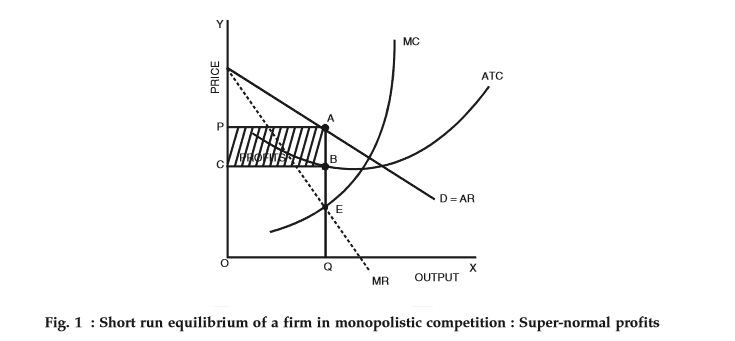# Price Determination of Firm and industry under Monopolistic competition

In monopolistic competition, since the product is differentiated between firms, each firm does not have a perfectly elastic demand for its products. In such a market, all firms determine the price of their own products. Therefore, it faces a downward sloping demand curve. Overall, we can say that the elasticity of demand increases as the differentiation between products decreases.Fig. 1 above depicts a firm facing a downward sloping, but flat demand curve. It also has a U-shaped short-run cost curve.

Conditions for the Equilibrium of an individual firm

The conditions for price-output determination and equilibrium of an individual firm are as follows:

1. MC = MR
2. The MC curve cuts the MR curve from below.

In Fig. 1, we can see that the MC curve cuts the MR curve at point E. At this point,

• Equilibrium price = OP and
• Equilibrium output = OQ

Now, since the per unit cost is BQ, we have

• Per unit super-normal profit (price-cost) = AB or PC.
• Total super-normal profit = APCB

The following figure depicts a firm earning losses in the short-run.From Fig. 2, we can see that the per unit cost is higher than the price of the firm. Therefore,

• AQ > OP (or BQ)
• Loss per unit = AQ – BQ = AB
• Total losses = ACPB

Long-run equilibrium

If firms in a monopolistic competition earn super-normal profits in the short-run, then new firms will have an incentive to enter the industry. As these firms enter, the profits per firm decrease as the total demand gets shared between a larger number of firms. This continues until all firms earn only normal profits. Therefore, in the long-run, firms, in such a market, earn only normal profits.As we can see in Fig. 3 above, the average revenue (AR) curve touches the average cost (ATC) curve at point X. This corresponds to quantity Q1 and price P1. Now, at equilibrium (MC = MR), all super-normal profits are zero since the average revenue = average costs. Therefore, all firms earn zero super-normal profits or earn only normal profits.

It is important to note that in the long-run, a firm is in an equilibrium position having excess capacity. In simple words, it produces a lower quantity than its full capacity. From Fig. 3 above, we can see that the firm can increase its output from Q1 to Q2 and reduce average costs. However, it does not do so because it reduces the average revenue more than the average costs. Hence, we can conclude that in monopolistic competition, firms do not operate optimally. There always exists an excess capacity of production with each firm.

In case of losses in the short-run, the firms making a loss will exit from the market. This continues until the remaining firms make normal profits only.#### intactone

View all posts by intactone →
error: Content is protected !!